# NCERT Solutions For Class 9 Maths Chapter 1 Exercise 1.3 – Number System

Download NCERT Solutions For Class 9 Maths Chapter 1 Exercise 1.3 – Number System. This Exercise contains 9 questions, for which detailed answers have been provided in this note. In case you are looking at studying the remaining Exercise for Class 9 for Maths NCERT solutions for Chapter 1 or other Chapters, you can click the link at the end of this Note.

### NCERT Solutions For Class 9 Maths Chapter 1 Exercise 1.3 – Number System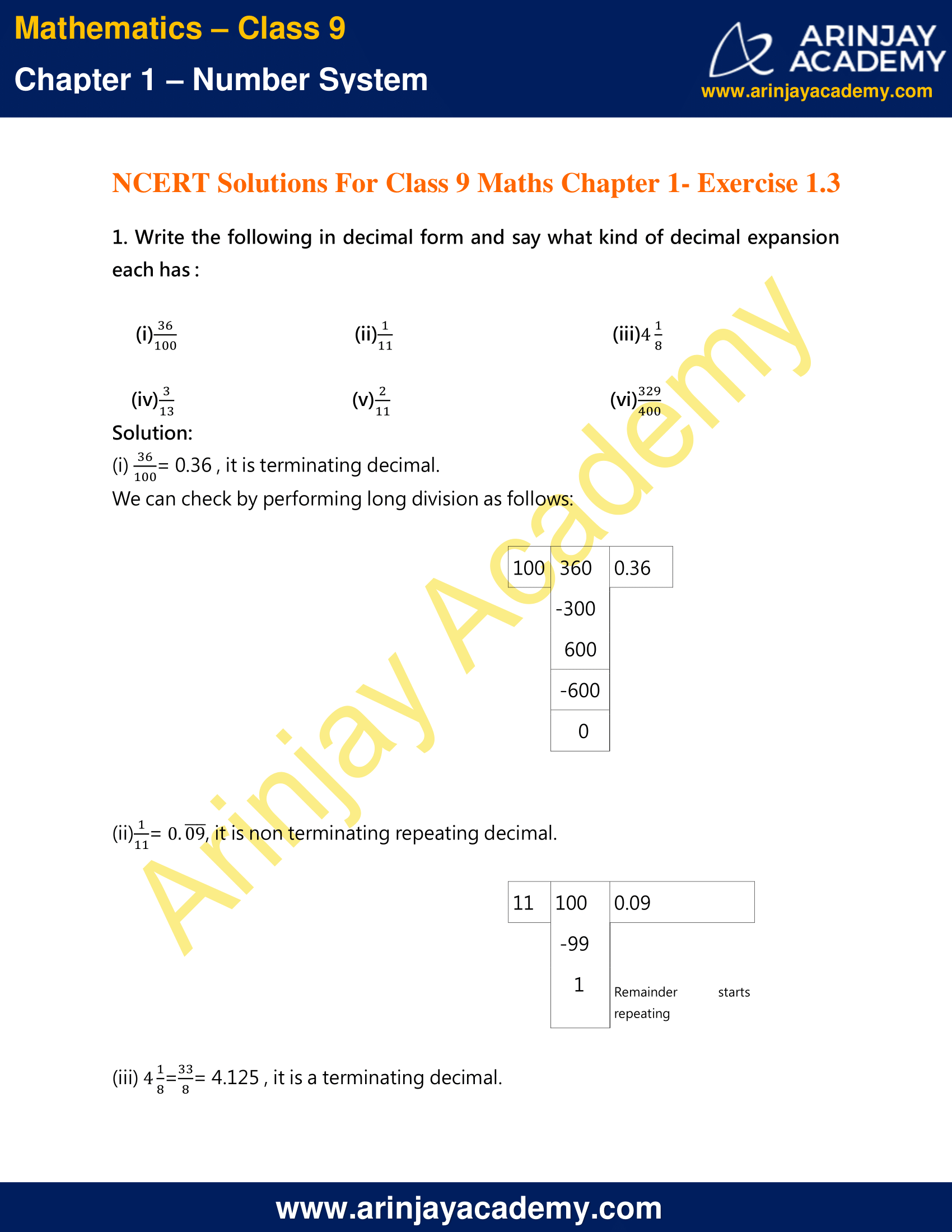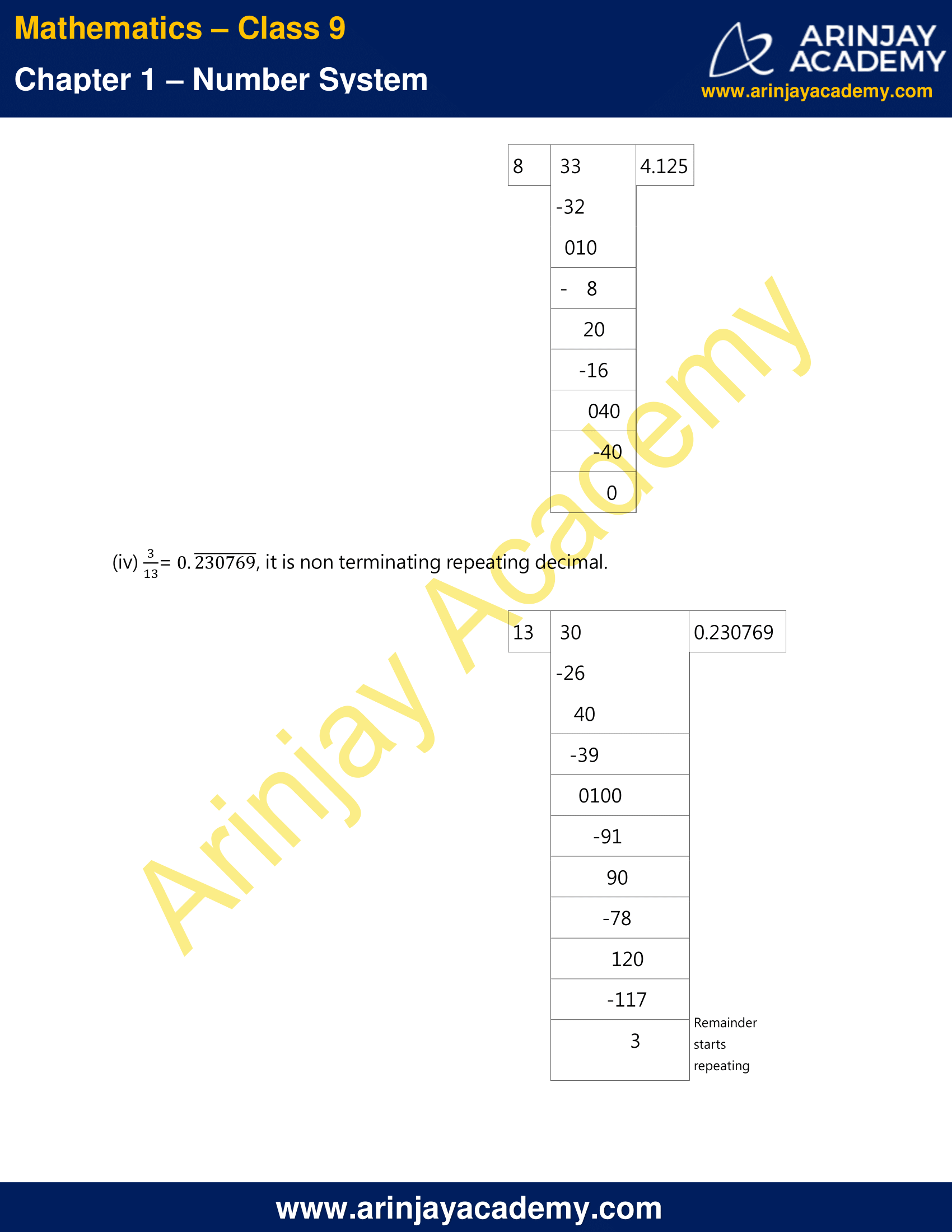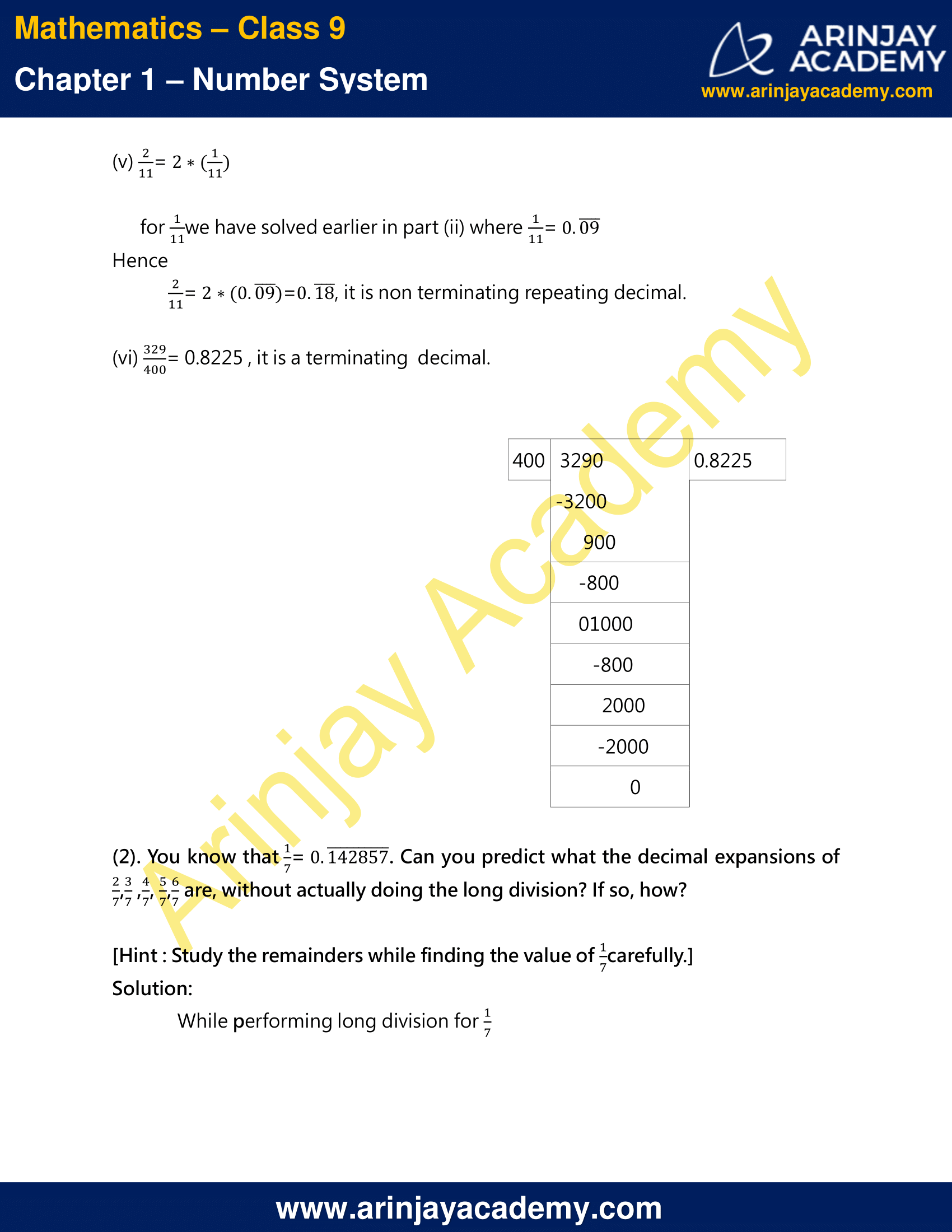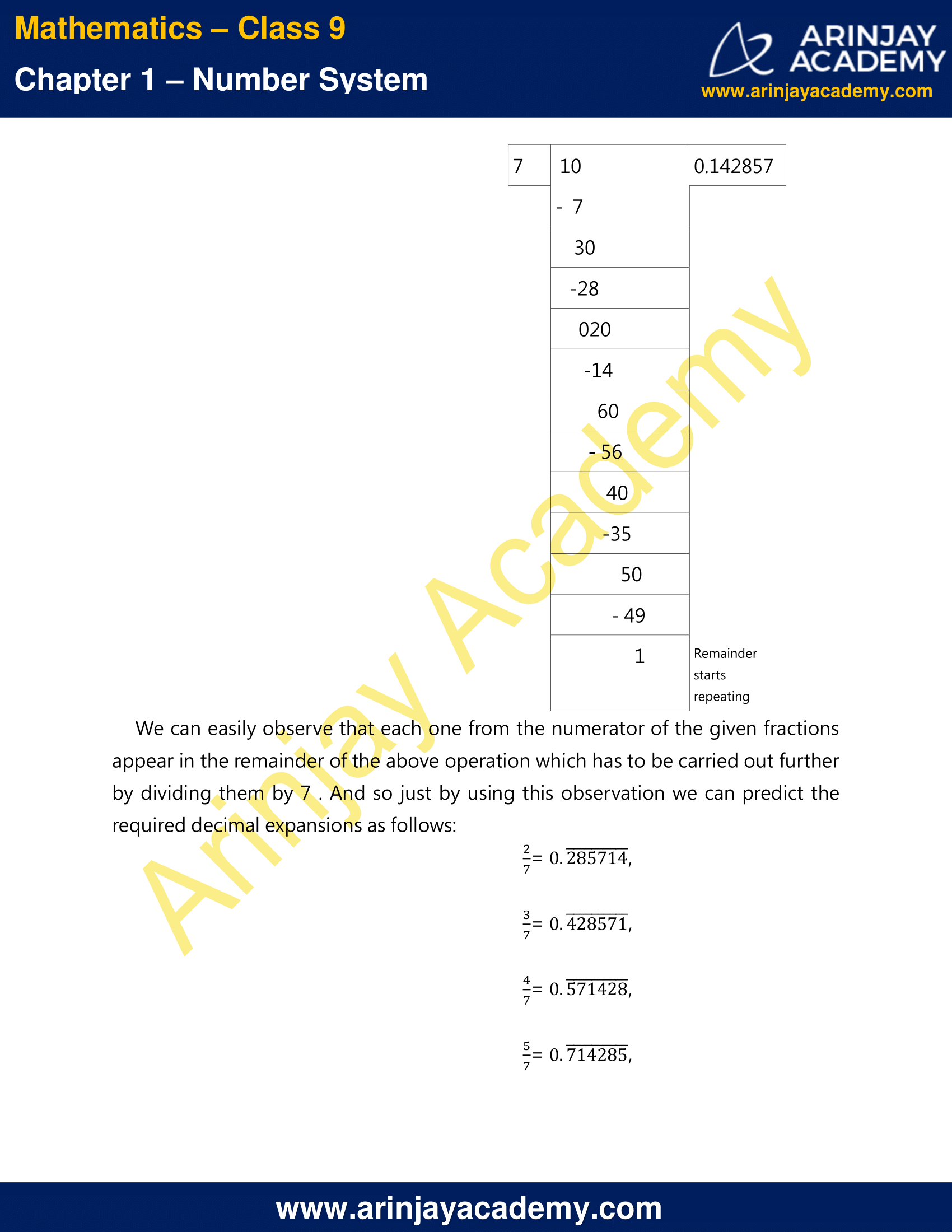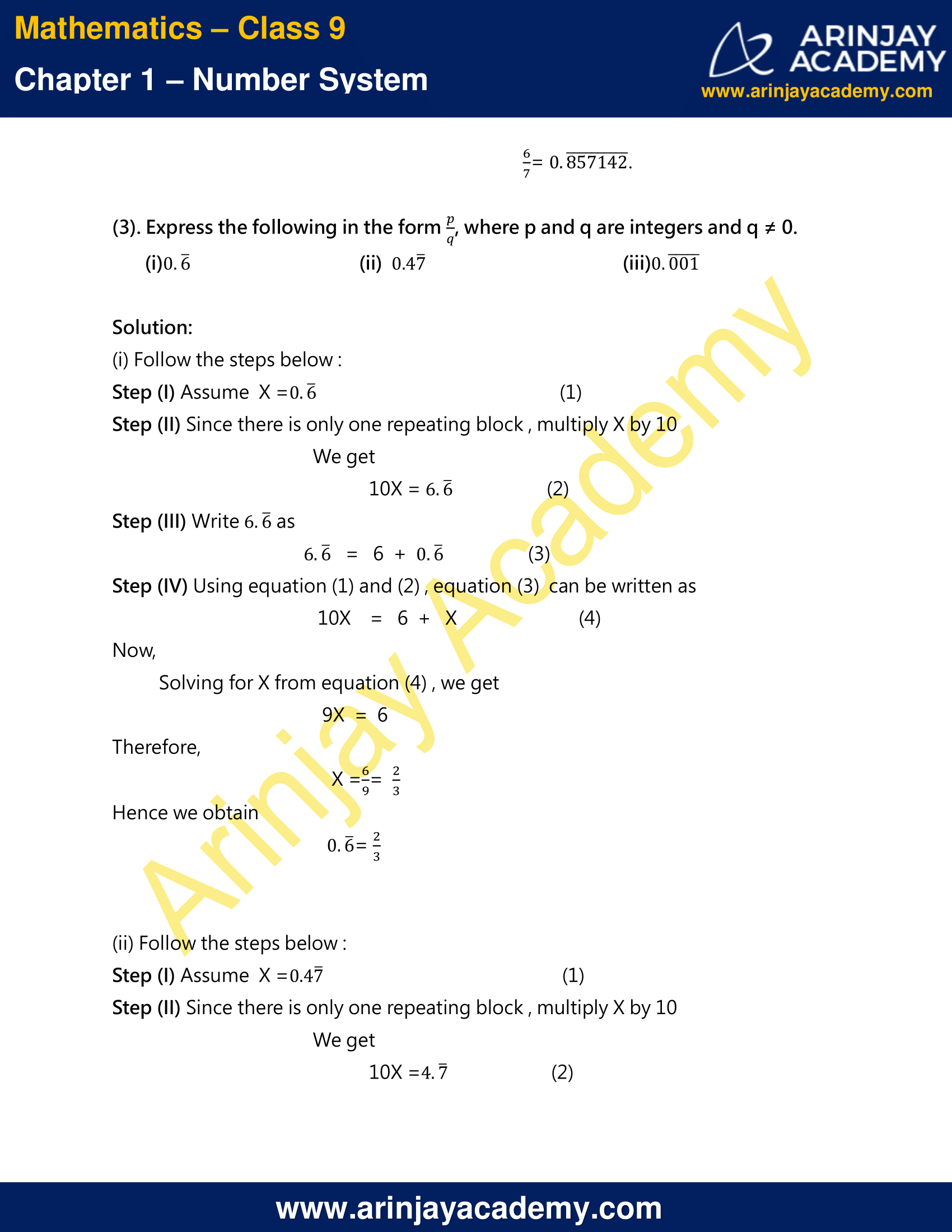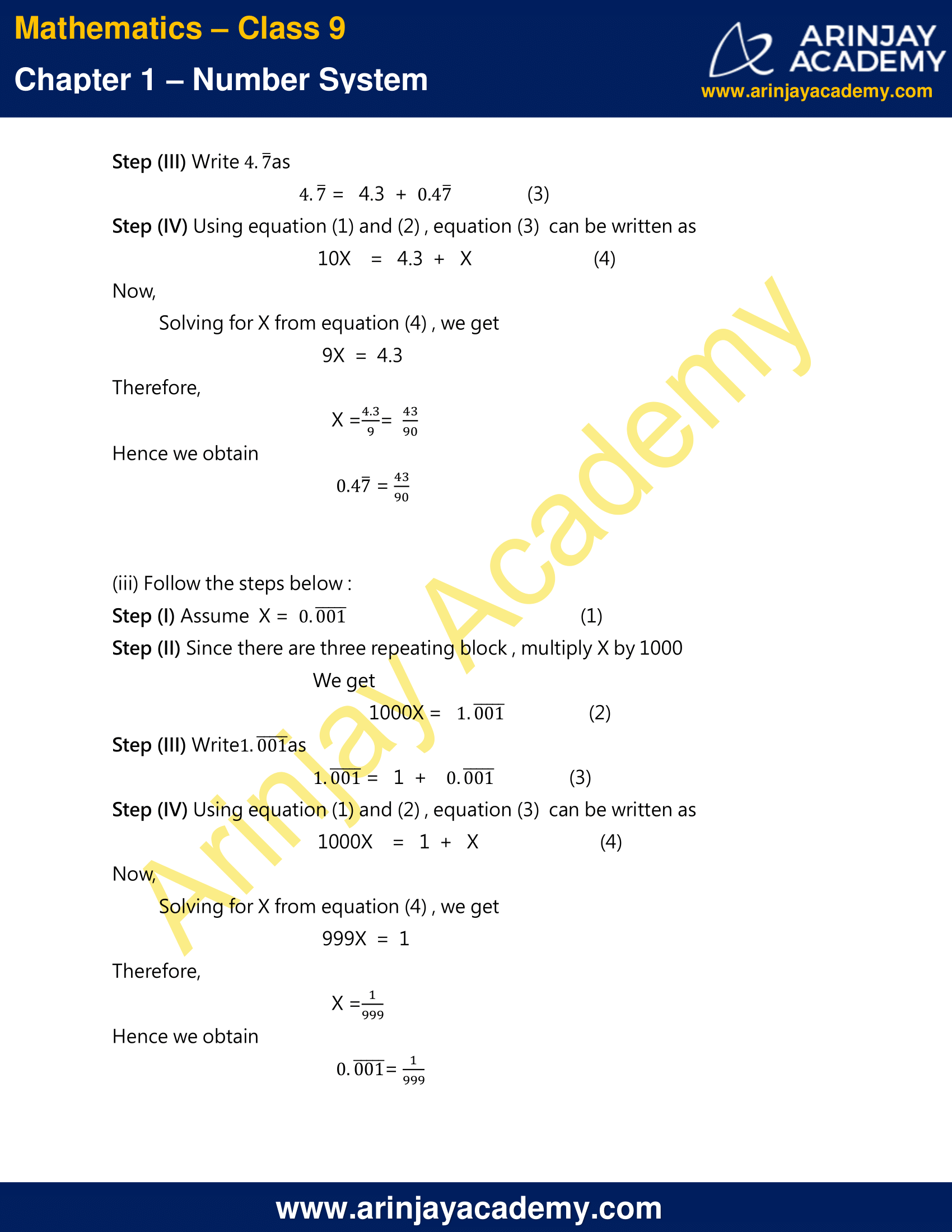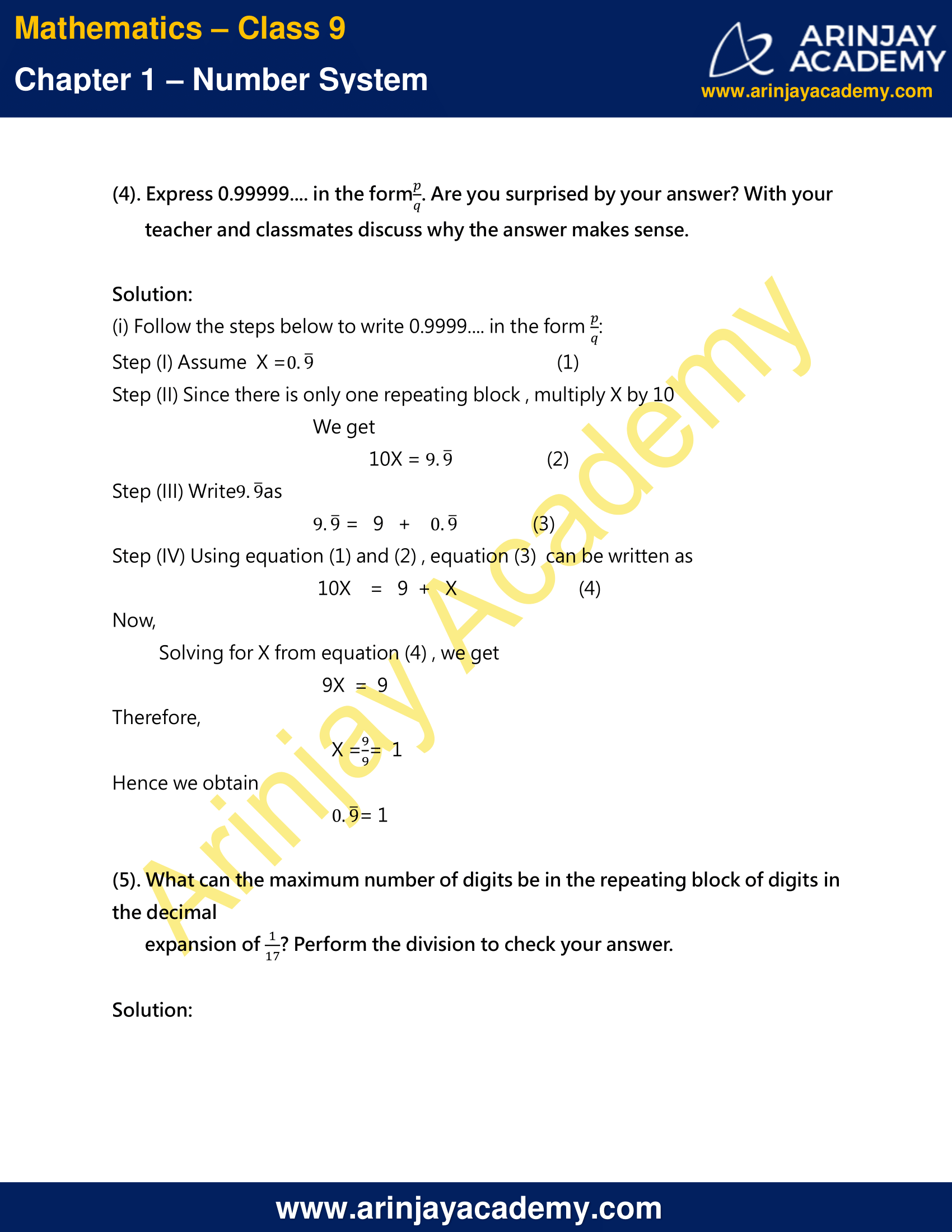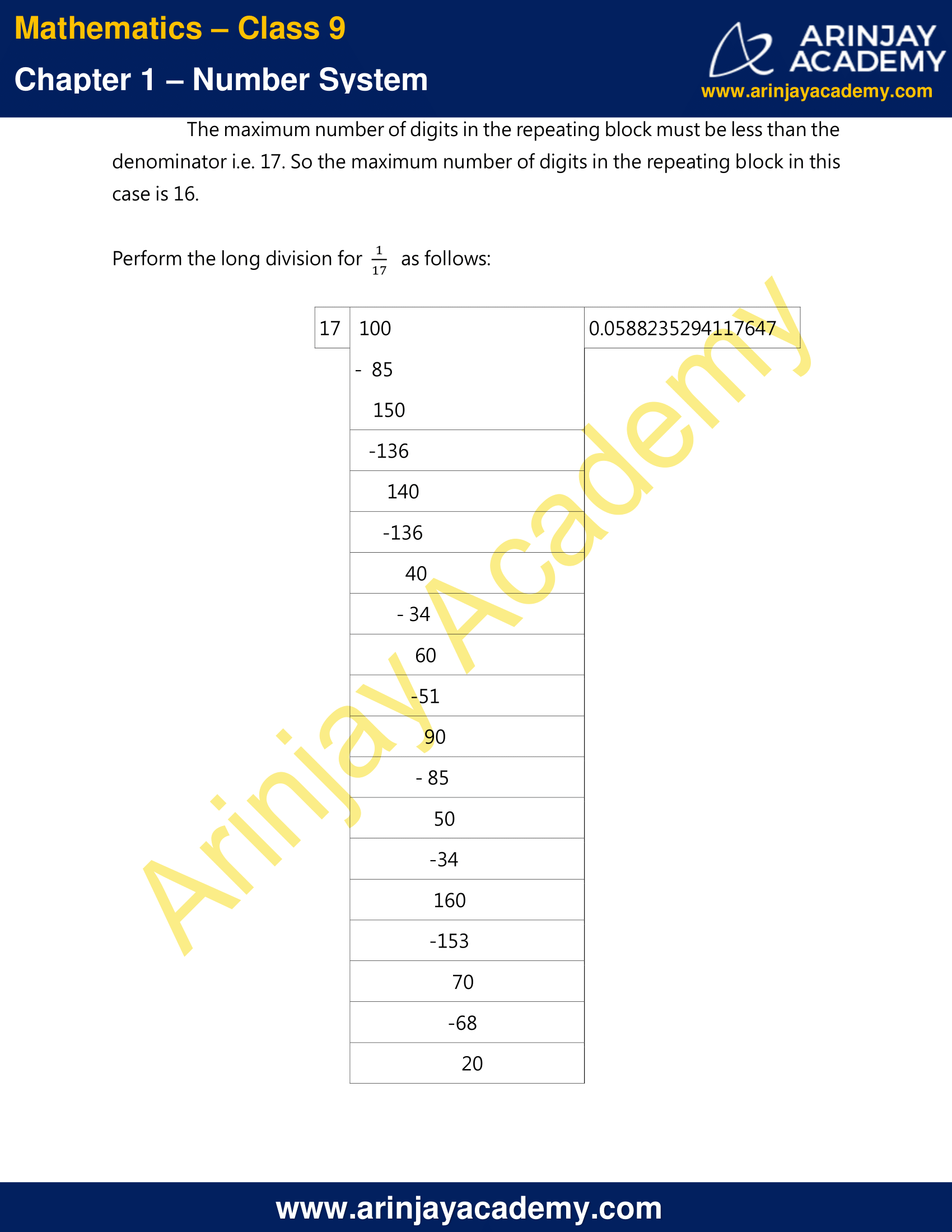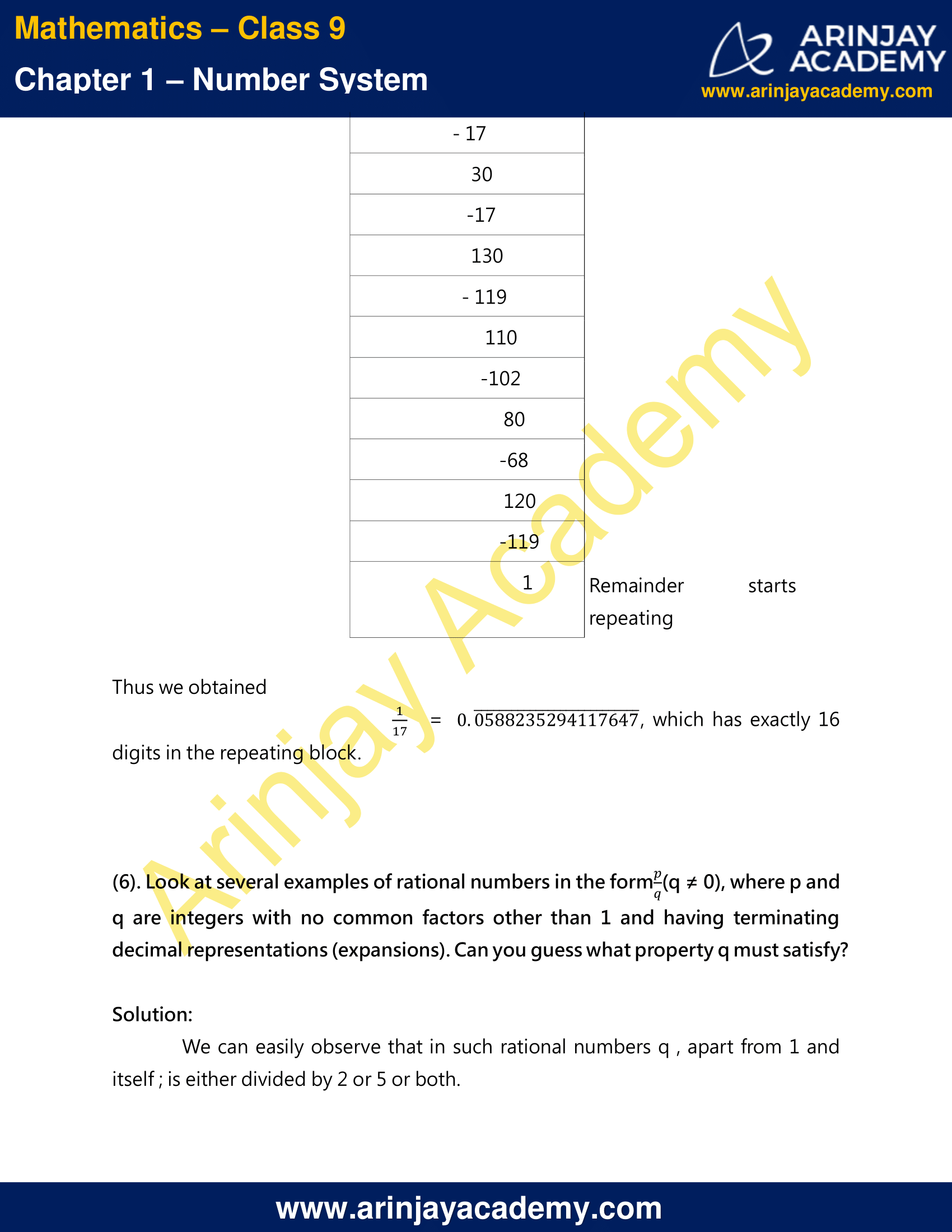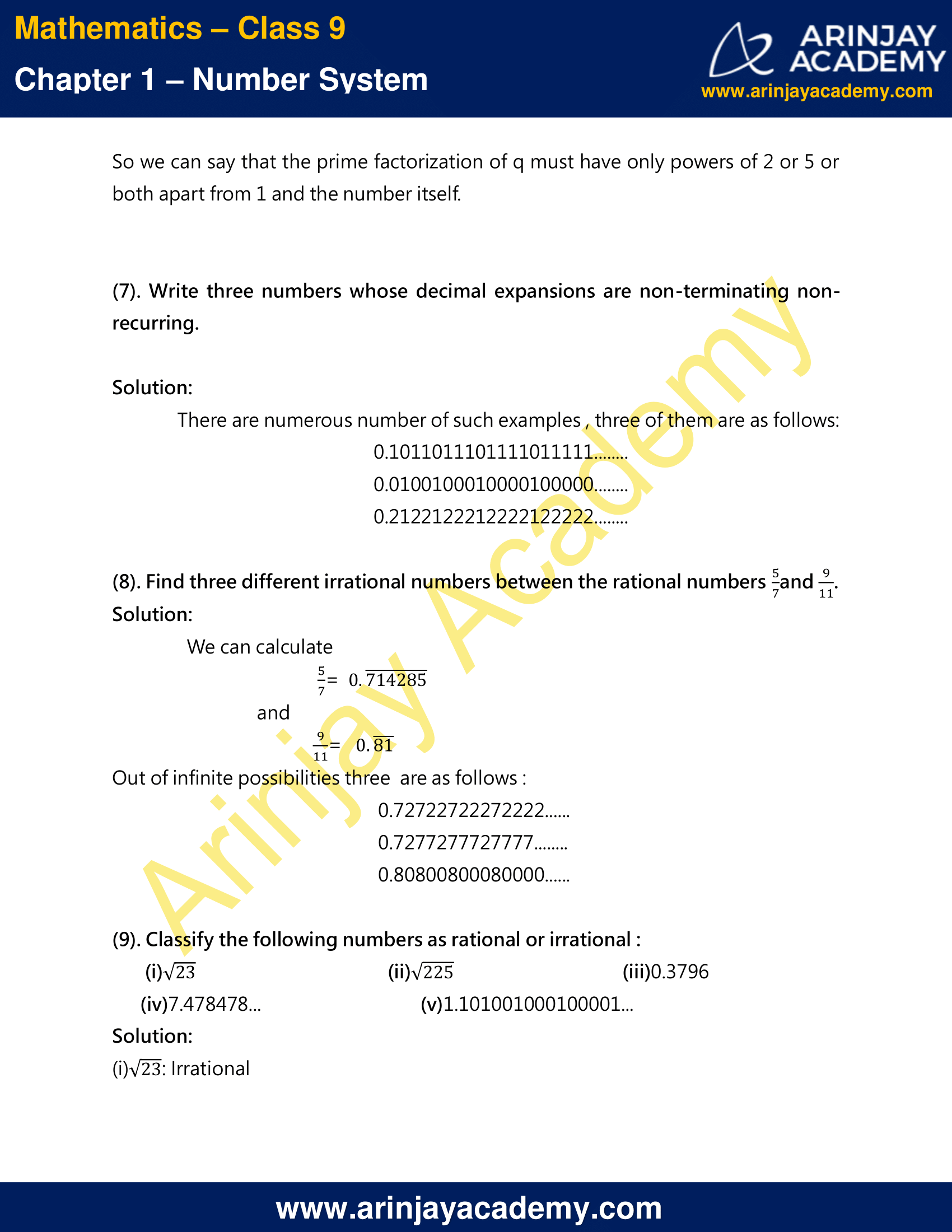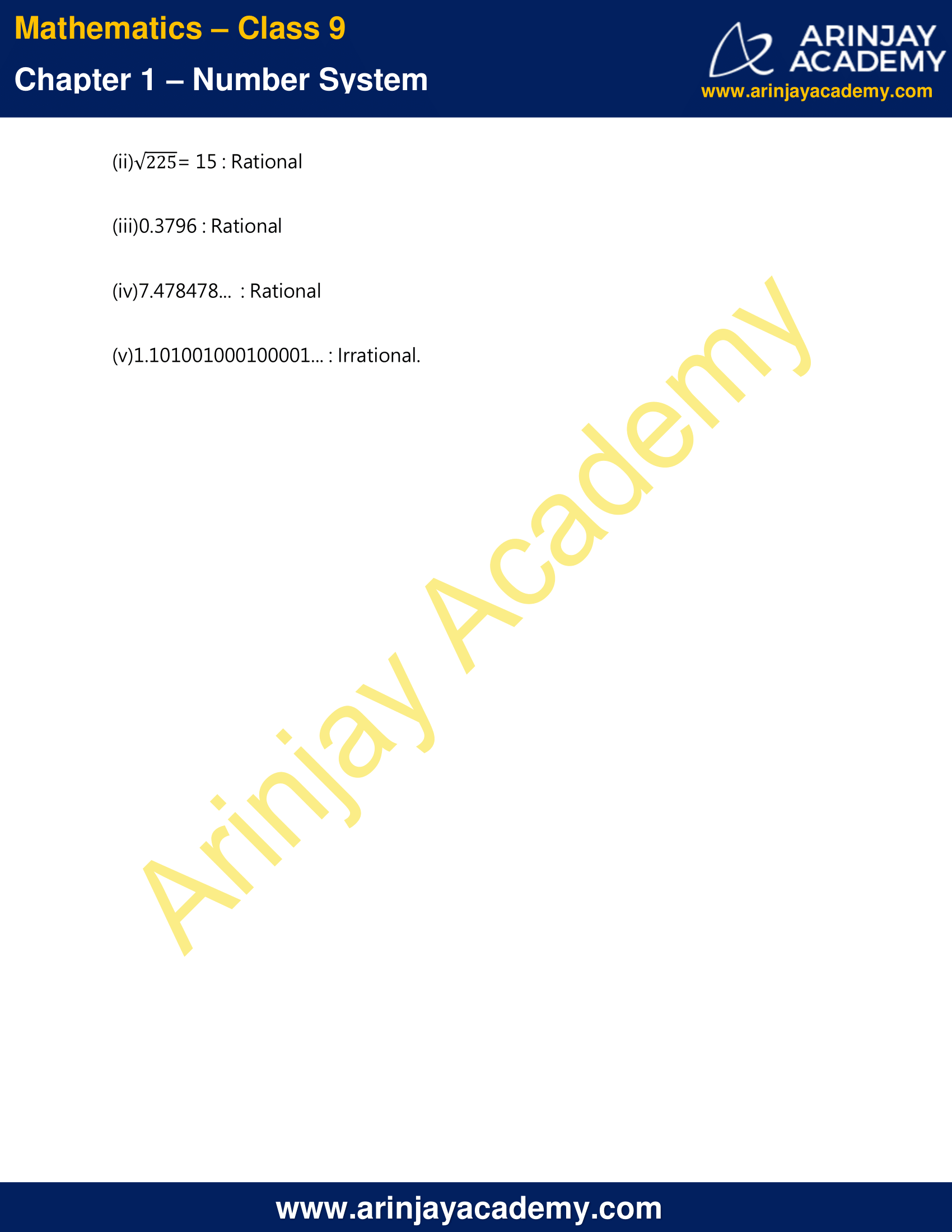NCERT Solutions For Class 9 Maths Chapter 1 Exercise 1.3 – Number System

1. Write the following in decimal form and say what kind of decimal expansion each has :

(i) 36/100

(ii) 1/11

(iii) 4 1⁄8

(iv) 3/13

(v) 2/11

(vi) 329/400

Solution:

(i) 36/100 = 0.36 , it is terminating decimal

We can check by performing long division as follows: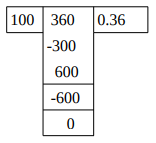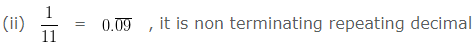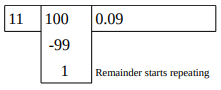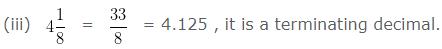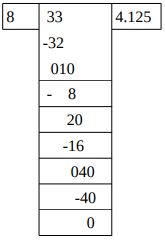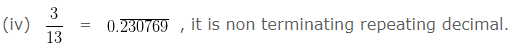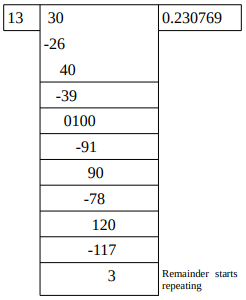(v) 2/11 = 2 x 1/11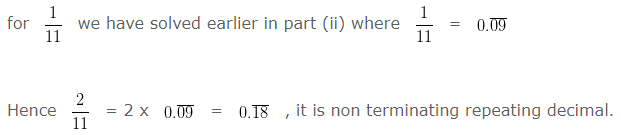(vi) 329/400 = 0.8225 , it is a terminating decimal.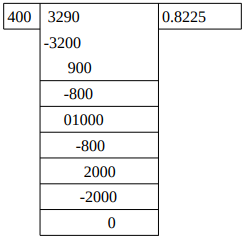(2). You know that 1/7 = 0.142857 . Can you predict what the decimal expansions of 2/7, 3/7, 4/7, 5/7, 6/7 are, without actually doing the long division? If so, how?

[Hint : Study the remainders while finding the value of 1/7 carefully.]

Solution:

While performing long division for 1/7.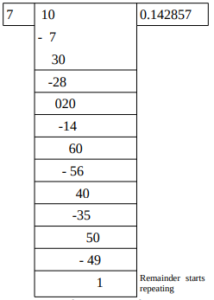We can easily observe that each one from the numerator of the given fractions appear in the remainder of the above operation which has to be carried out further by dividing them by 7 . And so just by using this observation we can predict the required decimal expansions as follows: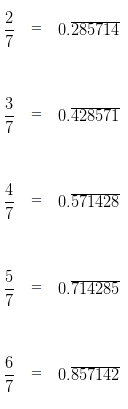(3). Express the following in the form p/q, where p and q are integers and q ≠ 0.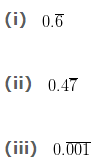Solution:

(i) Follow the steps below :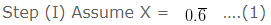Step (II) Since there is only one repeating block , multiply X by 10
We get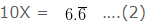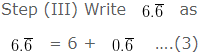Step (IV) Using equation (1) and (2) , equation (3) can be written as
10X = 6 + X ….(4)
Now,
Solving for X from equation (4) , we get
9X  =  6
Therefore,
X = 6/9 = 2/3
Hence we obtain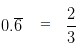(ii) Follow the steps below :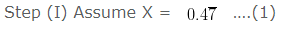Step (II) Since there is only one repeating block , multiply X by 10
We get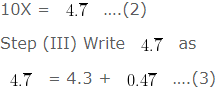Step (IV) Using equation (1) and (2) , equation (3) can be written as
10X = 4.3 + X  ….(4)
Now,
Solving for X from equation (4) , we get
9X  =  4.3
Therefore,
X = (4.3/9) = (43/90)
Hence we obtain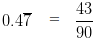(iii) Follow the steps below :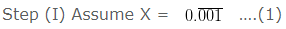Step (II) Since there are three repeating block , multiply X by 1000
We get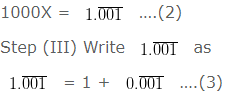Step (IV) Using equation (1) and (2) , equation (3) can be written as
1000X = 1 + X  ….(4)
Now,
Solving for X from equation (4) , we get
999X = 1
Therefore,
X = (1/999)
Hence we obtain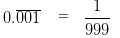(4). Express 0.99999…. in the form p/q . Are you surprised by your answer? With your teacher and classmates discuss why the answer makes sense.

Solution:

(i) Follow the steps below to write 0.9999…. in the form p/q: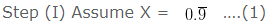Step (II) Since there is only one repeating block , multiply X by 10
We get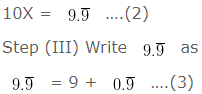Step (IV) Using equation (1) and (2) , equation (3) can be written as
10X = 9 + X ….(4)
Now,
Solving for X from equation (4) , we get
9X = 9
Therefore,
X = 9/9 = 1
Hence we obtain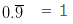(5). What can the maximum number of digits be in the repeating block of digits in the decimal expansion of 1/17 ? Perform the division to check your answer.

Solution:
The maximum number of digits in the repeating block must be less than the denominator i.e. 17. So the mximum number of digits in the repeating block in this case is 16.
Perform the long division for 1/17 as follows: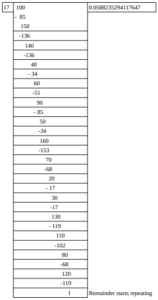Thus we obtained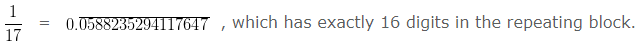(6). Look at several examples of rational numbers in the form p/q (q ≠ 0), where p and q are integers with no common factors other than 1 and having terminating decimal representations (expansions). Can you guess what property q must satisfy?

Solution:
We can easily observe that in such rational numbers q , apart from 1 and itself ; is either divided by 2 or 5 or both.
So we can say that the prime factorization of q must have only powers of 2 or 5 or both apart from 1 and the number itself.

(7). Write three numbers whose decimal expansions are non-terminating non-recurring.

Solution:

There are numerous number of such examples , three of them are as follows:
0.1011011101111011111……..
0.0100100010000100000……..
0.2122122212222122222……..

### Find three irrational numbers between given numbers – Video Explanation

(8). Find three different irrational numbers between the rational numbers 5/7 and 9/11.
Solution:

We can calculate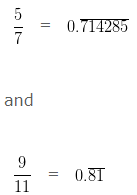Out of infinite possibilities three are as follows :
0.72722722272222……
0.7277277727777……..
0.80800800080000……

(9). Classify the following numbers as rational or irrational :
(i) √23
(ii) √225
(iii) 0.3796
(iv) 7.478478…
(v) 1.101001000100001…

Solution:

(i) √23 : Irrational
(ii) √225 = 15 : Rational
(iii)0.3796 : Rational
(iv)7.478478… : Rational
(v)1.101001000100001… : Irrational.

NCERT Solutions for Class 9 Maths Chapter 1 Exercise 1.3 – Number System, has been designed by the NCERT to test the knowledge of the student on the topic – Real Numbers and their Decimal Expansions

The next Exercise for NCERT Solutions for Class 9 Maths Chapter 1 Exercise 1.4 – Number System can be accessed by clicking here.

Download NCERT Solutions For Class 9 Maths Chapter 1 Exercise 1.3 – Number System